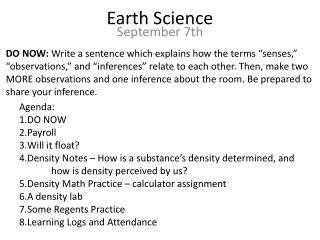DownloadDownload PresentationEarth Science

# Earth Science

Télécharger la présentation## Earth Science

- - - - - - - - - - - - - - - - - - - - - - - - - - - E N D - - - - - - - - - - - - - - - - - - - - - - - - - - -
##### Presentation Transcript

1. DO NOW: Write a sentence which explains how the terms “senses,” “observations,” and “inferences” relate to each other. Then, make two MORE observations and one inference about the room. Be prepared to share your inference. Earth Science • Agenda: • DO NOW • Payroll • Will it float? • Density Notes – How is a substance’s density determined, and how is density perceived by us? • Density Math Practice – calculator assignment • A density lab • Some Regents Practice • Learning Logs and Attendance September 7th

2. Density is… • …a number which represents the ratio of an object’s mass to its volume. • …a number which represents how heavy something is for its size. • Mass/Volume • Mass is the ‘heaviness’ of a substance, or how much matter it has. • Volume is the ‘bigness’ of a substance, or how much space it takes up.

3. Would the density of an object be high, or low? • …if an object were large, but didn’t weigh much? • Examples? • …if an object were small, but weighed a lot. • Examples?

4. The math of it… If you have two of the numbers, you can solve for the third. Use the density triangle, if you’re bad at math.

5. Practice makes permanent! On the sheet of lined paper you got, we’re going to practice some density problems. Take this opportunity seriously and ask for help if you need it. Before each problem, copy the density triangle. DON’T FORGET UNITS.

6. Let’s practice a few… Mass = 40 grams Volume = 40 milliliters Density = ? 40 g = = 1.0 g/mL 40 mL This is water! Liquid water has a density of about 1.0 g/mL. REMEMBER!

7. Let’s practice a few… Mass = 80 grams Volume = 40 milliliters Density = ? 80 g 2 g = = = 2.0 g/mL 40 mL 1 mL Is this substance more dense, less dense, or as dense as water? What would happen if you put this substance in water?

8. Let’s practice a few… Mass = 60 grams Volume = 120 milliliters Density = ? 60 g 1 g = = = .5 g/mL 120 mL 2 mL Is this substance more dense, less dense, or as dense as water? What would happen if you put this substance in water?

9. Let’s practice a few… Mass = ? grams Volume = 10 cm3 Density = 5.0 g/cm3 = = 5.0 g/cm3 X 10 cm3 m = D X v 50.0 grams